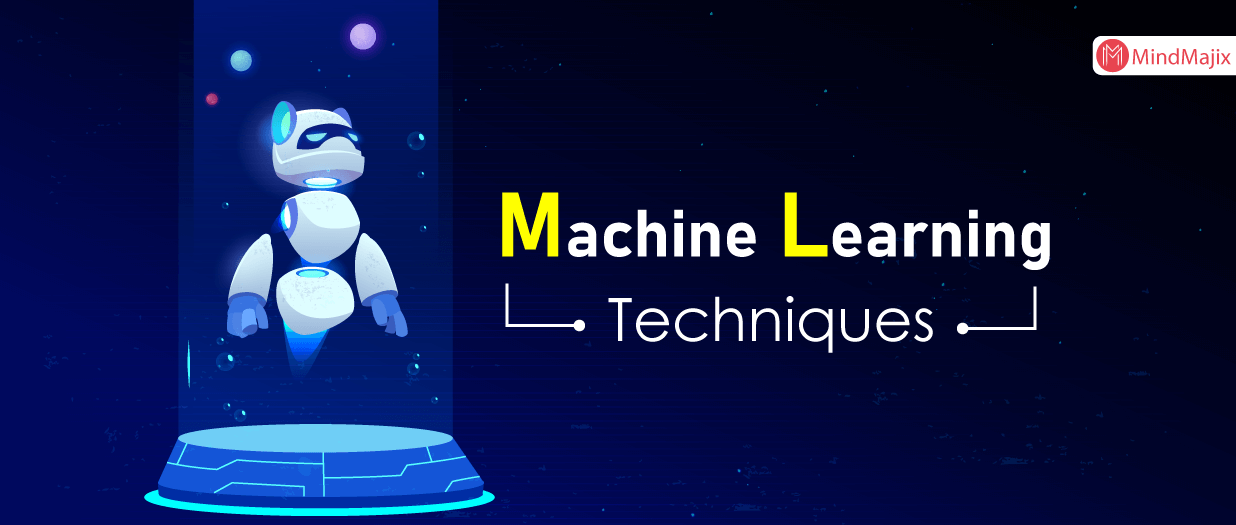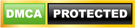Blog

# Machine Learning Techniques

• ###### | 1976 RatingsTechnology is something that has become an integral part of our day to day lives. Since from the past few decades it has been evolving to new heights to ease up the things. Technological disruption is taking everywhere. Machine learning is treated as one of the disruptive technologies of recent years.

Machine learning is a subfield of AI (Artificial Intelligence). It provides the ability to the systems to develop a capability to learn on their own and from the experiences they come across. The core idea behind machine learning is to design computer programs that let the machines to access the data and learn from it on their own.

Developing machines ability to learn on their own is a bit difficult task because it is associated with a lot of technical aspects. The process of learning consists of data or observation such as from experience, or instruction or with the examples that we give.  Once the machine is trained it understand the situations and provide the answers based on the data it has learned from. Machine learning enables computers to learn automatically and adjust according to conditions without the intervention of human beings.

We know that Machine learning largely depends on working with algorithms. There are various types of algorithms available in the market. Before getting to know them to let’s understand what is an algorithm is actually?

## What is an Algorithm?

An algorithm is defined as a set of rules and instructions to be followed while doing calculations or in problem-solving situations by a computer program.

An algorithm is a sequence of instructions to solve a particular problem. The common applications of algorithms are data processing, calculations, other computer, and mathematical operations. An algorithm contains a series of information to execute a task in a particular way.

Get ahead in your career by learning Machine Learning through Mindmajix Machine Learning Training.

## Decide Which Machine Learning Algorithm to Use?

Different organizations have different needs that are solved by machine learning algorithms. There is nothing like one solution or approach that can fit to your needs. There are multiple factors to be considered before selecting a machine learning algorithm.

There are many algorithms, and each one is specialised in solving a particular need. For example, if you wish to have a recommender type of solution, it's a common type of algorithm used to solve a specific problem. In the same fashion, some other problems are open and require a trial and error approach. Classification, regression, and supervised learning etc., are the algorithms which are able to solve the open and trial or error kind of problems.

Before searching for which algorithm to choose, it is better to identify and have a clear understanding of your problems and constraints. Let's consider some factors that could narrow down your search for which algorithm to choose.

### Factors to be considered :

Sometimes the selection of algorithms depends on the data we have. Algorithms works based on data, while some algorithms work with the only few samples whereas others require a ton and tons of data. So it is clear that specific algorithms works on certain types of data.

So it is quite essential to know your data:

1) look at the summary of data: Such as range of the data, Central Tendency, and identify the strong relationships.

2) Visualize data: Such as identifying the outliers, spread of data, and bivariate relationships etc.

• Find the missing value. As it affects the models more than anything else.
• Decide how to handle outliers.
• Take a decision on whether the data need to be aggregated or not.

Feature engineering makes your data ready for modelling. It serves for following

• Reduces data redundancy and dimensionality.
• Capture more complex relationships
• Ease up the models to interpret
• Rescues variables.

### Categorize the problem :

Categorizing problem is essential, and it is a two-step process

1) Categorize by input:

• If your data is labelled, it's a supervised learning problem.
• If it is unlabeled and wants to have a structure, then it is an unsupervised problem.
• It is considered as a reinforcement learning problem when you wish an objective function to interact with an environment.

2) Categorize by output:

• If the output of your model is a class, then it is a classification problem.
• If the output of your model is number, then it is a regression problem.
• If you want to detect an anomaly, then it is anomaly detection.
• It is considered as a clustering problem if your output model is a set of input groups.

• Being aware of your data storage capacity is quite essential to know before selecting a model. Some models such as regression/classification models require gigabytes of storage capability.
• What if the prediction required instantly? Let's say the autonomous cars need to be fast enough to act because it has to take the immediate movies based on the signs on the roads to avoid the accidents.
• Does the learning to be fast? There are some situations where training models require very fastly.  Sometimes you need to update the data models on the fly mode with a new dataset.

Now you have clear Idea on these on following things.

1. A better idea about what type of data you have
3. What constraints do you have
4. What are the data categorizations?

Now it's your turn to decide the various algorithms that are suitable for fulfilling your business needs.

Lets list out different types of algorithms used by machine learning.

Below mentioned are the commonly used machine learning algorithms.

• Linear regression
• Logistic regression
• Decision Tree
• SVM (support vector machine)
• Naive Bayes
• kNN
• K-Means
• Random Forest
• Dimensionality reduction algorithms
• GBM
• XGBoost
• Light GBM
• CatBoost

### Conclusion:

Algorithms are the main pillars of machine learning. Data plays a vital role in feeding the algorithms. Choosing the right data model may be a challenging task, but it full fills the solutions that you are looking for. It's very vital to conduct a right amount of research because once you select the model, it is going to show a major influence on your regular conduct of business.